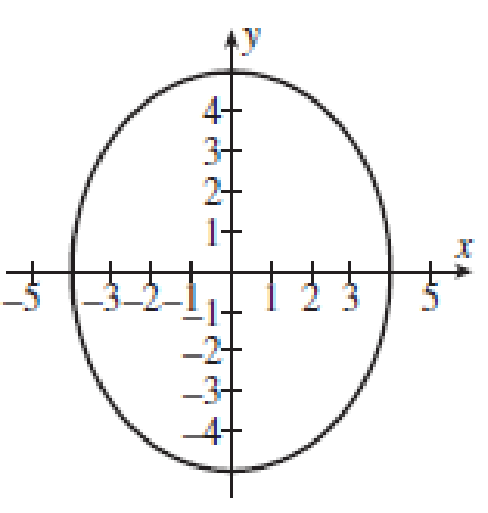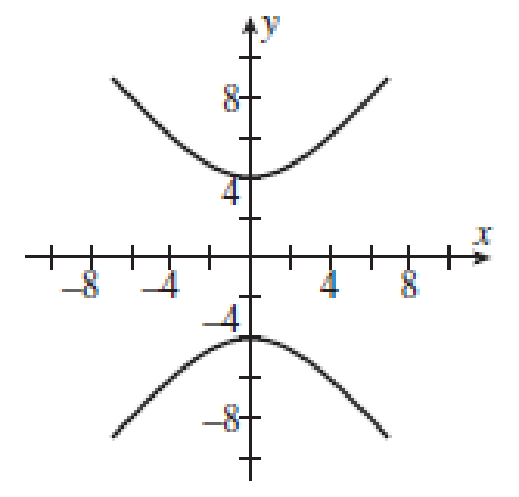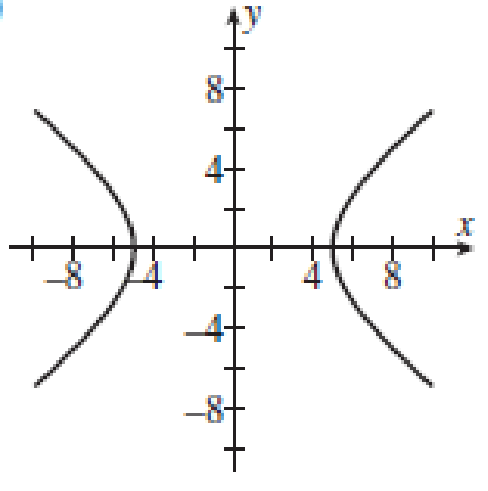Chapter 10.5, Problem 1PT

Chapter
Section
Textbook Problem

The graph of y 2 16 = 1 + x 2 25 is:a)b)c)d)To determine

To identify: The graph of y216=1+x225.

Explanation

Formula used:

The equation of the hyperbolas are: x2a2y2b2=1 with foci (±c,0), where c2=a2+b2, and vertices (±a,0) and y2a2x2b2=1 with foci (±c,0), where c2=a2+b2, and vertices (0,±a).

Calculation:

The given equation is, y216=1+x225

Still sussing out bartleby?

Check out a sample textbook solution.

See a sample solution

The Solution to Your Study Problems

Bartleby provides explanations to thousands of textbook problems written by our experts, many with advanced degrees!

Get Started

Sometimes, Always, or Never: If an ≥ bn ≥ 0 for all n and {bn} diverges, then {an} diverges.

Study Guide for Stewart's Single Variable Calculus: Early Transcendentals, 8th

Solve the equations in Exercises 112 for x (mentally, if possible). 4x5=8

Finite Mathematics and Applied Calculus (MindTap Course List)

Find the critical numbers of the function. f(x) = x2e3x

Single Variable Calculus: Early Transcendentals, Volume I

In Exercises 7-10, find the slope of the line shown in each figure. 8.

Applied Calculus for the Managerial, Life, and Social Sciences: A Brief Approach

In Problems 5-10, use integration to find the general solution to each differential equation.

Mathematical Applications for the Management, Life, and Social Sciences

Find the seventh term of the arithmetic progression x, x+y,x+2y,.....

Finite Mathematics for the Managerial, Life, and Social Sciences

Construct a truth table for the given statement. p(qp)

Mathematical Excursions (MindTap Course List)